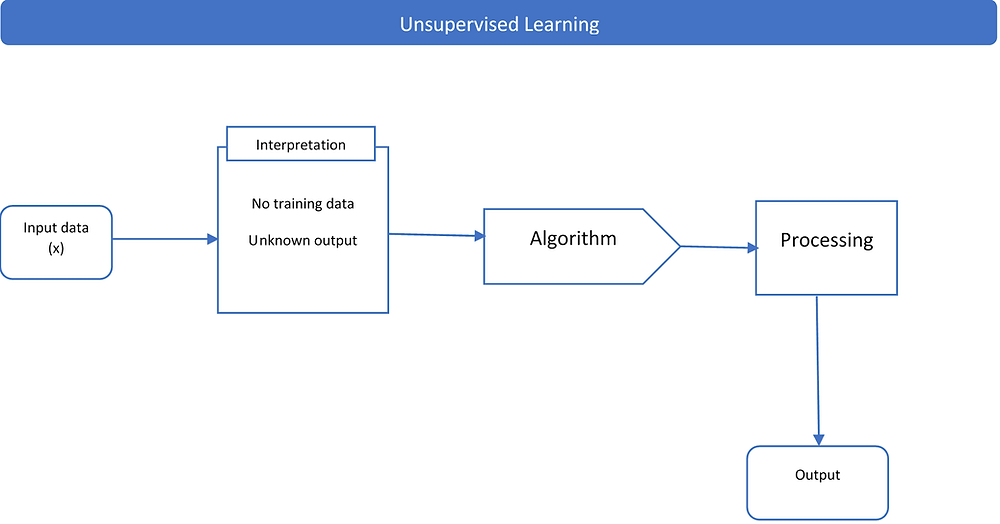Search

# Machine Learning - What is it?

A baby learns to crawl, walk and then run.  We are in the crawling stage when it comes to applying machine learning. -Dave WatersMachine learning (ML) is the science of getting computers to use data and information to learn and improve their knowledge over time.

The machines go through an automatic learning process, consisting of observation of historical data, identifying patterns that predict future observations, and ultimately support the decision-making process.

## I. Types of Machine Learning

The basis of ML is ‘Learning”. The mainly used types of learning include:

## 1. Supervised machine learningIt is the process of using algorithms to learn by mapping an input (x) to an output (Y) to achieve a high level of prediction (Y) with new input data (x).

It is denominated supervised because this is like having a teacher supervising the process to ensure the degree of prediction.

Supervised learning problems include:

· Classification: When the output variables are a category, such as “Active” or “Lost to follow-up” and “dead”.

· Regression: When the output variable is a numeric value such as “Weight” or “Retention rate”.

## 2. Unsupervised machine learningIt is the process of using algorithms to learn more about the data. Here the input data (x) is available, and there is no corresponding output variable (Y).

It is unsupervised because there is no wrong or right answer, and there is no teacher. Algorithms explore, learn and present the structure in the data.

Conventional unsupervised methods are:

· Clustering: used to discover groupings

· Association: used to establish association among variables inside large databases.

### 3. Semi-Supervised LearningA reality found on many of the real-world datasets; it is the process of using algorithms to learn the mapping of input (x) to an output (Y) where just some of the data is labeled (Y).

In this method, unsupervised learning algorithms are used to explore the input variables(x), and supervised learning algorithms are used to make predictions to the unlabeled data (Y) and then use the data back in the supervised algorithm as training data to predict new data.

## 4. Reinforcement LearningIt is a learning method where the algorithms try to continually find the best possible solution or path for a current situation. It differs from the other methods as there is no training data with input (x) and output (Y) variables. Here the algorithm learns sequentially from its own experience.

In reinforcement learning, the algorithm interacts with its environments, meaning that the output depends on the state of the current input and the next input depends on the output of the previous input.